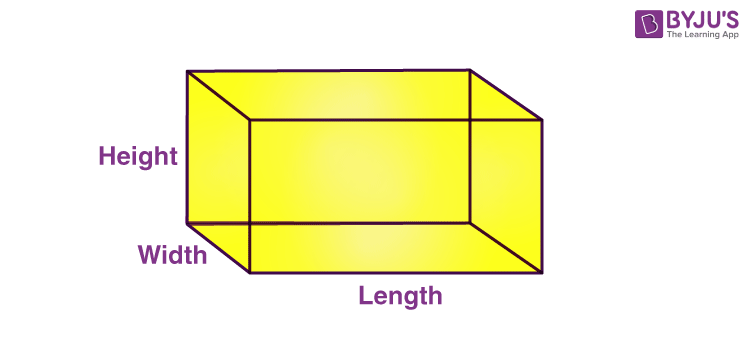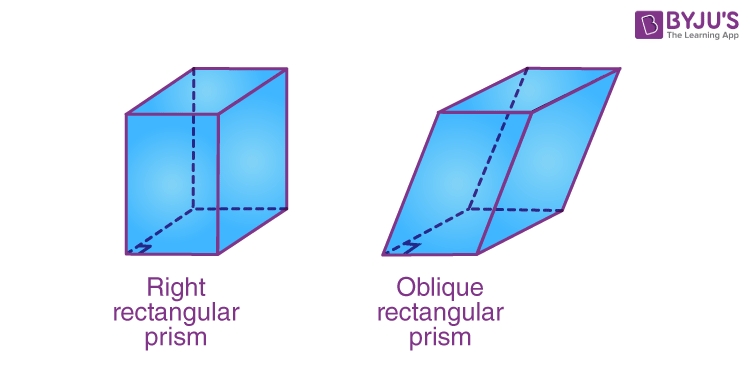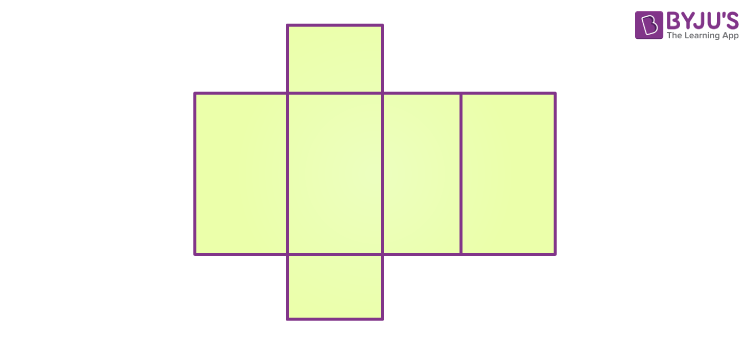# Rectangular Prism

In geometry, a rectangular prism is a polyhedron with two congruent and parallel bases. It is also called a cuboid. A rectangular prism has six faces, and all the faces are in a rectangle shape and have twelve edges. Because of its cross-section along the length, it is said to be a prism. Geometry is the study of shapes and the configuration of objects. Similar to other three-dimensional shapes, a rectangular prism also has its surface area and volume. The surface area of the prism is the area of its net. In this article, let us discuss the definition, types, surface area, and volume of a rectangular prism in detail.

## What is a Rectangular Prism?

A rectangular prism is a three-dimensional shape, that has six faces (two at the top and bottom and four are lateral faces). All the faces of the prism are rectangular in shape. Hence, there are three pairs of identical faces here. Due to its shape, a rectangular prism is also called a cuboid. Some of the real-life examples of a rectangular prism are a geometry box, notebooks, diaries, rooms, etc. In the below figure, we can see the shape of a rectangular prism.## Properties of Rectangular Prism

• A rectangular prism has 6 faces, 12 edges and 8 vertices
• The top and base of the rectangular prism are always a rectangle
• Like cuboid, it also has three dimensions, i.e., length width and height
• Pairs of opposite faces are identical or congruent
• For a right rectangular prism, the lateral faces are rectangle
• For an oblique rectangular prims, the lateral faces are parallelogram
• It has a rectangular cross-section
• It looks exactly like a cuboid

## Types of Rectangular Prism

Rectangular prism can be classified into two different types. They are:

1. Right Rectangular Prism
2. Oblique Rectangular Prism

### Right Rectangular Prism

A prism with rectangular bases is called a rectangular prism. A right rectangular prism is a prism that has six faces that are rectangles, and all angles are right angles.

• Vertices of a rectangular prism = 8
• Edges of a rectangular prism = 12
• Faces of a rectangular prism= 6 (including bases)

### Oblique Rectangular prism

An oblique prism is a prism in which the bases are not perpendicular to each other. A rectangular prism with bases that are not aligned one directly above the other is an oblique rectangular prism.## Rectangular Prism Formulas

A rectangular prism is a three-dimensional object. Hence, it will have its surface area and volume. To calculate the volume and surface area of a prism, we have to know the length of its sides or edges. Let ‘l’, ‘w’ and ‘h’ be the length, width and height of the rectangular prism. The formulas are given below.

### Volume of a Rectangular Prism  Formula

The volume of a rectangular prism is a measurement of the occupied units of a rectangular prism. The volume of a rectangular prism is represented by cubic units. It is also defined as the number of units used to fill a rectangular prism.

The volume of the rectangular prism is equal to the area of the base times its height.

Therefore, the volume of a rectangular prism formula is given as

The volume of a rectangular prism = Length x Width x Height cubic units.

 Volume = l x w x h cubic units

### Surface Area of a Rectangular Prism

The surface area of a rectangular prism is the measure of how much-exposed area a prism has. Surface area is expressed in square units. The total surface area of a rectangular prism is the sum of the lateral surface area (LSA) and twice the base area of the rectangular prism.

Total Surface Area of rectangular prism = LSA + 2 (Base area)       [Square units]

The lateral surface area of a rectangular prism is the sum of the surface area of all its faces without the base of the rectangular prism. The lateral surface area of any right rectangular prism is equivalent to the perimeter of the base times the height of the prism.

Therefore, the lateral surface area = P x h [Square units]

Where

P is the perimeter of a base

h be the height of the prism

The perimeter of the rectangular prism is,

 P = 2 (l + w)

Therefore, the lateral surface area (LSA) of a rectangular prism = 2 ( l + w ) h square units.

Hence,

TSA = LSA + 2 (Base Area) = 2 (l + w) h + 2 (l x w) = 2 lh + 2 wh + 2 lw   [Square units]

Therefore, the surface area of a rectangular prism formula is given as,

 Surface Area of a rectangular prism = 2 (lh +wh + lw ) Square units.

## Rectangular Prism Net

The net of any prism is its surface area. It shows when we open the prism in a plane; then all its sides could be visible at the same time. If we calculate the individual area of all its sides using the net, we will get the total surface area. See the figure below to find the net for the rectangular prism.You can see from the above figure, all the sides of the prism are in a rectangular shape. By using the formula for the area of rectangle, you can find the areas for each face and add all the areas to get the net of the prism.

## Solved Examples on Rectangular Prism

Question 1: Find the volume of a rectangular prism whose length, width, and height are 8cm, 6cm, and 4cm, respectively.

Solution:

Given:

Length, l = 8 cm

Width, w = 6 cm

Height, h = 4 cm

The formula to find the volume of a rectangular prism is,

V = Length x Width x Height cubic units

V = 8 x 6 x 4 cm3

V = 192 cm3

Therefore, the volume of a rectangular prism is 192 cm3.

Question 2: Find the surface area of a rectangular prism whose length, width, and height are 8cm, 6cm, and 4cm, respectively.

Solution: Given:

Length, l = 8 cm

Width, w = 6 cm

Height, h = 4 cm

The formula to find the area of a rectangular prism is,

A = 2 (lh +wh + lw )

A = 2 (8×4+6×4+8×6)

A = 2(32+24+48)

A = 2(104)

A = 208 sq.cm.

## Practice Questions

1. Find the area of a rectangular prism whose length, width, and height are given, respectively.

• 3cm, 4cm and 5cm
• 2.5 cm, 6cm, 9cm
• 5 cm, 8cm, 10cm
• 6.2 cm, 4.4 cm, 9cm

2. Find the volume of rectangular prism with the following dimensions.

• 3cm x 4cm x 5cm
• 2.5 cm x 6cm x 9cm
• 5 cm x 8cm x 10cm
• 6.2 cm x 4.4 cm x 9cm

Stay tuned with BYJU’S – The Learning App for more Maths-related concepts and download the app to watch personalized videos.

## Frequently Asked Questions on Rectangular Prism

Q1

### What is the right rectangular prism?

A right rectangular prism has 6 rectangular faces, 12 edges and 8 vertices. It is also called a cuboid.
Q2

### What is the difference between the right rectangular prism and an oblique rectangular prism?

The bases of the right rectangular prism are perpendicular to each other whereas the bases of the oblique rectangular prism are not perpendicular.
Q3

### What is an example of a rectangular prism?

The rectangular prism examples in real life are bricks, books, doors, etc.
Q4

### What is the volume of a rectangular prism?

The volume of a rectangular prism is equal to the product of its length, width and height.
Q5

### What is the surface area of a rectangular prism?

The surface area of a rectangular prism is given by:
SA = 2 (lh +wh + lw ) Square units.
Q6

### What is the rule for rectangular prism?

The bases of rectangular prism (top and bottom) should be rectangular in shape.
Q7

### Is a rectangular prism also a cuboid?

A right rectangular prism has all its six faces, rectangular, similar to a cuboid. Hence it is also known as a cuboid.
Test your Knowledge on Rectangular Prism